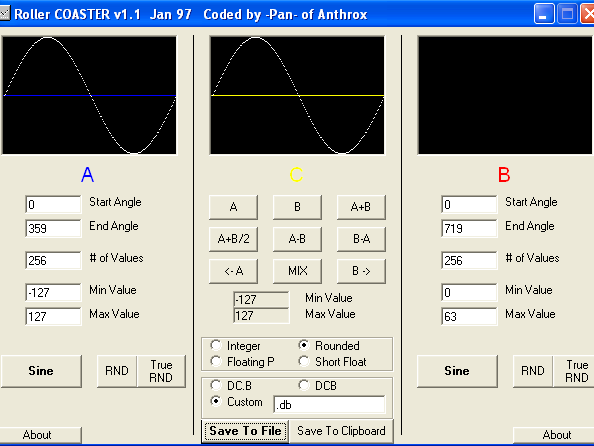You really need to know fixed point complementary math for this one. Now time for a trig overview. In the 'real' world, we have 360 degrees of rotation, and the results of sin and cos of any angle fall in the range of -1 to 1. Now this works a little differently on the SNES, at least the way I ended up doing it.

In the SNES world, we are going to represent the fractional values that range from -1 to +1 as -127 to +127. Also, instead of going through 360 values for 360 degrees we are going to cycle through 256 values that represent 360 degrees of rotation. Why? Because we need to have a way of representing positive and negative fractional values and if we cycle through only 256 values we can use just 1 byte to do the cycling. Moreover, The complementary math registers we'll be using to do the calculations need 1 of the multiplicands to be only a byte in size. So now we can multiply our scaling factors by an angle and do both scaling & rotation!

Now we're left with the question, how are we going to obtain a table of 256 values that represents sin and cos results from -127 to +127? I found a program called RollerCoaster, made by Pan. It's Windows only :(, but that's what we have visualization for now :D. Mac or Linux will need visualization. Anyhow, grab RollerCoaster.7z. Now take a look below:Enter in all the values listed under A, then in the middle column labeled C, press the A button. This will copy our A sinusoid into C, which is used for output into a lookup table. Lastly, make sure the 'rounded' bullet is selected as well as the 'Custom' bullet. Specify '.db' as the custom manner to define bytes in the lookup table. We do this of course because WLA uses the .db directive to define bytes. Now click 'Save To File' and name it '`sincos.inc`'.

Once we have a sin lookup table we can index into it and obtain any value for sin and cos. We can obtain any cos value since the cos is just a horizontal shift of sin. `cos(θ) = sin(θ + 90)` I converted this from 'real life' to my SNES version by knowing that this equation is done for a range of 360 values, and 90 is 360/4. Now we have 256 values, and 256/4 = 64, thus: `cos(θ) = sin(θ + 64)`

Now you need to learn how to do signed complementary math on the SNES. Multiple registers are used. It basically multiplies a number 16-bits wide by an 8-bit wide number, and the result can be anywhere from 8-24 bits in size. Let me show you the registers for the multiplicands:

``````Register \$211B: Multiplicand A (W/2b)
Register \$211C: Multiplicand B (W/1b)

Register \$2134-\$2136: Result (R/3b)
``````

We're going to be using a 8.8 fixed number for multiplicand A, which will be the scale value. Multiplicand B is going to be 0.8 fixed number (only the fraction). An 8.8 · 0.8 = 8.16. This means that `\$2136` will be holding the whole number while `\$2135` will have the upper half of the fractional value and `\$2134` the lower part. When we get the answer we'll only be concerned with the whole number and upper fractional result, so we'll just be reading from `\$2135-\$2136`.

Angle Calculations

``````θ: Rotation angle
α: X scale factor
β: Y scale factor

[ A  B ] = [ cos(θ)·(1/α)  -sin(θ)·(1/α) ]
[ C  D ]   [ sin(θ)·(1/β)   cos(θ)·(1/β) ]
``````

Note: Rotation is counter-clockwise. For clockwise rotation, you need to put a -sin in C and +sin in B.

VBlank is the only good time to use the multiplication registers since `\$211B-\$211C` are being used for the Mode7 matrix parameters at any other time. Here's how to perform the calculations during vblank:

``````VBlank:
REP #\$30
LDA angle
TAX

; Calculate B and C (the sin's)
; B
LDA sx            ; scale_x low byte
STA \$211B         ; Multiplicand A low byte
LDA sx+1          ; scale_x high byte
STA \$211B         ; Multiplicand A high byte
LDA sincos,X      ; sin(x)
EOR #\$FF          ; Make negative
INA               ; Make negative
STA \$211C         ; Multiplicand B
LDY \$2135         ; Result -> 8.8
STY B
; C
LDA sy            ; scale_y low byte
STA \$211B         ; Multiplicand A low byte
LDA sy+1          ; scale_y high byte
STA \$211B         ; Multiplicand A high byte
LDA sincos,X      ; sin(x)
STA \$211C         ; Multiplicand B
LDY \$2135         ; Result -> 8.8
STY C

; Change X index to point to cos values
TXA               ; X index to A
CLC               ; clear carry
TAX               ; A to X index

; Calculate A and D (the cos's)
; A
LDA sx            ; scale_x low byte
STA \$211B         ; Multiplicand A low byte
LDA sx+1          ; scale_x high byte
STA \$211B         ; Multiplicand A high byte
LDA sincos,X      ; cos(x)
STA \$211C         ; Multiplicand B
LDY \$2135         ; Result -> 8.8
STY A
; D
LDA sy            ; scale_y low byte
STA \$211B         ; Multiplicand A low byte
LDA sy+1          ; scale_y high byte
STA \$211B         ; Multiplicand A high byte
LDA sincos,X      ; cos(x)
STA \$211C         ; Multiplicand B
LDY \$2135         ; Result -> 8.8
STY D

; Store results as Matrix Parameters
LDA A
STA \$211B
LDA A+1
STA \$211B

LDA B
STA \$211C
LDA B+1
STA \$211C

LDA C
STA \$211D
LDA C+1
STA \$211D

LDA D
STA \$211E
LDA D+1
STA \$211E

RTI

sincos:
.include "sincos.inc"
``````

Complete Source Code: mode-7-rotate-tutorial.7z

Tutorial by bazz